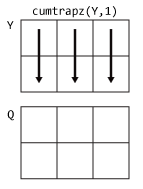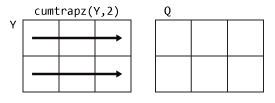# cumtrapz

Cumulative trapezoidal numerical integration

## Syntax

``Q = cumtrapz(Y)``
``Q = cumtrapz(X,Y)``
``Q = cumtrapz(___,dim)``

## Description

example

````Q = cumtrapz(Y)` computes the approximate cumulative integral of `Y` via the trapezoidal method with unit spacing. The size of `Y` determines the dimension to integrate along:If `Y` is a vector, then `cumtrapz(Y)` is the cumulative integral of `Y`.If `Y` is a matrix, then `cumtrapz(Y)` is the cumulative integral over each column.If `Y` is a multidimensional array, then `cumtrapz(Y)` integrates over the first dimension whose size does not equal 1.```

example

````Q = cumtrapz(X,Y)` integrates `Y` with respect to the coordinates or scalar spacing specified by `X`. If `X` is a vector of coordinates, then `length(X)` must be equal to the size of the first dimension of `Y` whose size does not equal 1.If `X` is a scalar spacing, then `cumtrapz(X,Y)` is equivalent to `X*cumtrapz(Y)`. ```

example

````Q = cumtrapz(___,dim)` integrates along the dimension `dim` using any of the previous syntaxes. You must specify `Y`, and optionally can specify `X`. If you specify `X`, then it can be a scalar or a vector with length equal to `size(Y,dim)`. For example, if `Y` is a matrix, then `cumtrapz(X,Y,2)` cumulatively integrates each row of `Y`.```

## Examples

collapse all

Calculate the cumulative integral of a vector where the spacing between data points is 1.

Create a numeric vector of data.

`Y = [1 4 9 16 25];`

`Y` contains function values for $\mathit{f}\left(\mathit{x}\right)={\mathit{x}}^{2}$ in the domain `[1 5]`.

Use cumtrapz to integrate the data with unit spacing.

`Q = cumtrapz(Y)`
```Q = 1×5 0 2.5000 9.0000 21.5000 42.0000 ```

This approximate integration yields a final value of 42. In this case, the exact answer is a little less, $41\frac{1}{3}$. The `cumtrapz` function overestimates the value of the integral because f(x) is concave up.

Calculate the cumulative integral of a vector where the spacing between data points is uniform, but not equal to 1.

Create a domain vector.

`X = 0:pi/5:pi;`

Calculate the sine of `X`.

`Y = sin(X');`

Cumulatively integrate `Y` using `cumtrapz`. When the spacing between points is constant, but not equal to 1, an alternative to creating a vector for `X` is to specify the scalar spacing value. In that case, `cumtrapz(pi/5,Y)` is the same as `pi/5*cumtrapz(Y)`.

`Q = cumtrapz(X,Y)`
```Q = 6×1 0 0.1847 0.6681 1.2657 1.7491 1.9338 ```

Cumulatively integrate the rows of a matrix where the data has a nonuniform spacing.

Create a vector of x-coordinates and a matrix of observations that take place at the irregular intervals. The rows of `Y` represent velocity data, taken at the times contained in `X`, for three different trials.

```X = [1 2.5 7 10]; Y = [5.2 7.7 9.6 13.2; 4.8 7.0 10.5 14.5; 4.9 6.5 10.2 13.8];```

Use `cumtrapz` to integrate each row independently and find the cumulative distance traveled in each trial. Since the data is not evaluated at constant intervals, specify `X` to indicate the spacing between the data points. Specify `dim = 2` since the data is in the rows of `Y`.

`Q1 = cumtrapz(X,Y,2)`
```Q1 = 3×4 0 9.6750 48.6000 82.8000 0 8.8500 48.2250 85.7250 0 8.5500 46.1250 82.1250 ```

The result is a matrix of the same size as `Y` with the cumulative integral of each row.

Perform nested integrations in the x and y directions. Plot the results to visualize the cumulative integral value in both directions.

Create a grid of values for the domain.

```x = -2:0.1:2; y = -2:0.2:2; [X,Y] = meshgrid(x,y);```

Calculate the function $\mathit{f}\left(\mathit{x},\mathit{y}\right)=10{\mathit{x}}^{2}+20{\mathit{y}}^{2}$ on the grid.

`F = 10*X.^2 + 20*Y.^2;`

`cumtrapz` integrates numeric data rather than functional expressions, so in general the underlying function does not need to be known to use `cumtrapz` on a matrix of data. In cases where the functional expression is known, you can instead use `integral`, `integral2`, or `integral3`.

Use `cumtrapz` to approximate the double integral

`$I\left(a,b\right)={\int }_{-2}^{b}{\int }_{-2}^{a}\left(10{x}^{2}+20{y}^{2}\right)\phantom{\rule{0.16666666666666666em}{0ex}}dx\phantom{\rule{0.16666666666666666em}{0ex}}dy.$`

To perform this double integration, use nested function calls to `cumtrapz`. The inner call first integrates the rows of data, then the outer call integrates the columns.

`I = cumtrapz(y,cumtrapz(x,F,2));`

Plot the surface representing the original function as well as the surface representing the cumulative integration. Each point on the surface of the cumulative integration gives an intermediate value of the double integral. The last value in `I` gives the overall approximation of the double integral, `I(end) = 642.4`. Mark this point in the plot with a red star.

```surf(X,Y,F,'EdgeColor','none') xlabel('X') ylabel('Y') hold on surf(X,Y,I,'FaceAlpha',0.5,'EdgeColor','none') plot3(X(end),Y(end),I(end),'r*') hold off```## Input Arguments

collapse all

Numeric data, specified as a vector, matrix, or multidimensional array. By default, `cumtrapz` integrates along the first dimension of `Y` whose size does not equal 1.

Data Types: `single` | `double`
Complex Number Support: Yes

Point spacing, specified as `1` (default), a uniform scalar spacing, or a vector of coordinates.

• If `X` is a scalar, then it specifies a uniform spacing between the data points and `cumtrapz(X,Y)` is equivalent to `X*cumtrapz(Y)`.

• If `X` is a vector, then it specifies x-coordinates for the data points and `length(X)` must be the same as the size of the integration dimension in `Y`.

Data Types: `single` | `double`

Dimension to operate along, specified as a positive integer scalar. If no value is specified, then the default is the first array dimension whose size does not equal 1.

Consider a two-dimensional input array, `Y`:

• `cumtrapz(Y,1)` works on successive elements in the columns of `Y`.• `cumtrapz(Y,2)` works on successive elements in the rows of `Y`.If `dim` is greater than `ndims(Y)`, then `cumtrapz` returns an array of zeros of the same size as `Y`.

## Tips

• Use `trapz` and `cumtrapz` to perform numerical integrations on discrete data sets. Use `integral`, `integral2`, or `integral3` instead if a functional expression for the data is available.

• `trapz` reduces the size of the dimension it operates on to 1, and returns only the final integration value. `cumtrapz` also returns the intermediate integration values, preserving the size of the dimension it operates on.